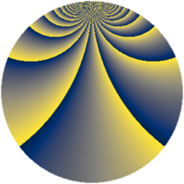# Properties

 Label 2001.2.zLevel $2001$ Weight $2$ Character orbit 2001.z Rep. character $\chi_{2001}(320,\cdot)$ Character field $\Q(\zeta_{22})$ Dimension $2240$ Sturm bound $480$

# Related objects

## Defining parameters

 Level: $$N$$ $$=$$ $$2001 = 3 \cdot 23 \cdot 29$$ Weight: $$k$$ $$=$$ $$2$$ Character orbit: $$[\chi]$$ $$=$$ 2001.z (of order $$22$$ and degree $$10$$) Character conductor: $$\operatorname{cond}(\chi)$$ $$=$$ $$69$$ Character field: $$\Q(\zeta_{22})$$ Sturm bound: $$480$$

## Dimensions

The following table gives the dimensions of various subspaces of $$M_{2}(2001, [\chi])$$.

Total New Old
Modular forms 2440 2240 200
Cusp forms 2360 2240 120
Eisenstein series 80 0 80

## Trace form

 $$2240q + 4q^{3} + 216q^{4} - 4q^{9} + O(q^{10})$$ $$2240q + 4q^{3} + 216q^{4} - 4q^{9} - 16q^{12} - 22q^{15} - 216q^{16} - 56q^{18} - 264q^{25} + 16q^{27} - 88q^{30} - 8q^{31} - 22q^{33} - 44q^{34} - 24q^{36} - 88q^{37} - 32q^{39} - 308q^{40} - 12q^{46} + 132q^{48} + 240q^{49} - 180q^{52} + 52q^{54} + 72q^{55} + 220q^{60} + 88q^{61} + 328q^{64} + 122q^{69} + 136q^{70} + 172q^{72} + 48q^{73} + 78q^{75} + 176q^{76} - 324q^{78} + 88q^{79} - 140q^{81} + 120q^{82} - 308q^{84} + 48q^{85} - 418q^{90} - 16q^{93} - 168q^{94} - 394q^{96} - 132q^{97} + O(q^{100})$$

## Decomposition of $$S_{2}^{\mathrm{new}}(2001, [\chi])$$ into newform subspaces

The newforms in this space have not yet been added to the LMFDB.

## Decomposition of $$S_{2}^{\mathrm{old}}(2001, [\chi])$$ into lower level spaces

$$S_{2}^{\mathrm{old}}(2001, [\chi]) \cong$$ $$S_{2}^{\mathrm{new}}(69, [\chi])$$$$^{\oplus 2}$$